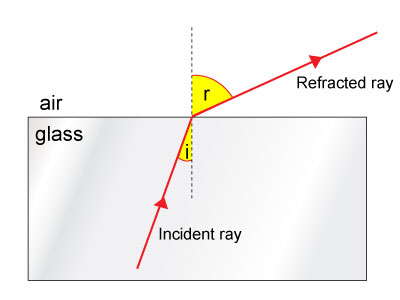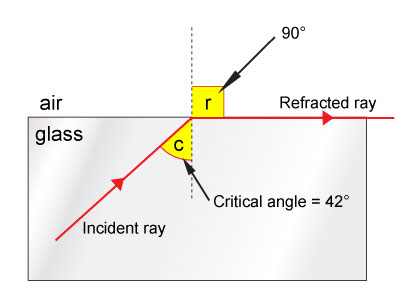## Total Internal Reflection

When light travels from one medium to another it changes speed and is refracted. If the light rays are travelling for a less dense material to a dense medium they are refracted towards the normal and if they are travelling from a dense to less dense medium they are refracted away from the normal.

For total internal reflection to occur the light must travel from a dense medium to a less dense medium (e.g. glass to air or water to air).

As the angle of incidence increases so does the angle of refraction. When the angle of incidence reaches a value known as the critical angle the refracted rays travel along the surface of the medium or in other words are refracted to an angle of 90°. The critical angle for the angle of incidence in glass is 42°.

1. Angle of incidence less than the critical angleWhen the angle of incidence of the light ray leaving the glass is less than the critical angle, the light ray speeds up on leaving the glass and is refracted away from the normal.
2. Angle of incidence equal to the critical angleWhen the angle of incidence of the light ray reaches the critical angle (42°) the angle of refraction is 90°. The refracted ray travels along the surface of the denser medium in this case the glass.

3. Angle of incidence greater than the critical angleWhen the angle of incidence of the light ray is greater than the critical angle then no refraction takes place. Instead, all the light is reflected back into the denser material in this case the glass. This is called total internal reflection.

In order for total internal reflection to take place:

1. The rays of light must travel from a dense medium to a less dense medium.
2. The angle of incidence must be greater than the critical angle.